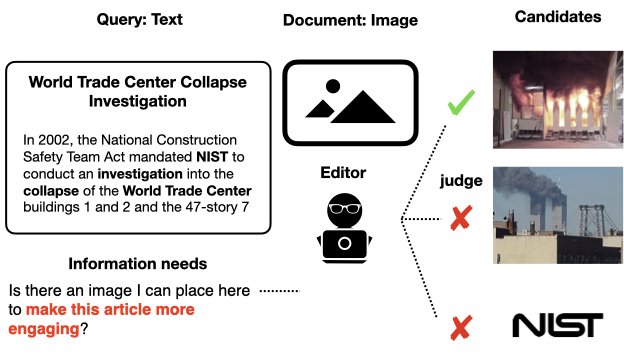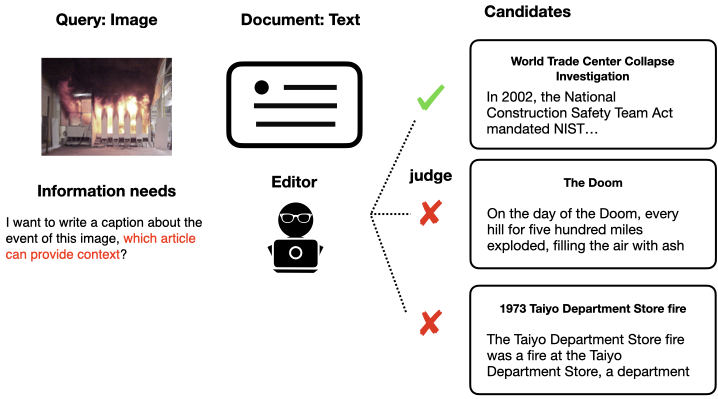# What is AToMiC?

See our white paper for more technical details.

Authoring Tools for Multimedia Content (AToMiC) Track at the Text Reterieval Conference (TREC) is intended to encourage research in multimedia search systems. We aim to provide evaluation resources and toolkits for researchers from information retrieval, natural language processing, computer vision, and multimedia communities.

## Motivation

Multimedia search is still a challenging task. The ever-growing production of text–image data has brought many works from different research communities to address the problem of effectively accessing multimedia resources. However, their capabilities for real-world applications is still questionable. The de facto image–text datasets (Flickr30k and MS-COCO) are constructed to generate image-text associations based on high-level concepts (i.e., describing generic objects such as mountain, or cat), while real-world multimedia search applications often deal with very specific semantic entities (e.g. Mount Fuji, or Larry the Cat). Hence, we need a better proxy to evaluate multimodal retrieval systems.

The TREC 2023 AToMiC track is organised with two tasks of the standard ad hoc retrieval setup. We assume the existence of a corpus $$\mathcal{C}$$ comprised of a collection of documents $$\{d_1, d_2 \ldots d_n\}$$. In response to a user’s information need represented as query $$q$$. The system’s goal is to return a top-$$k$$ ranked list of documents that maximizes some metric of quality such as nDCG or MRR.

For TREC 2023, we provide two different collections: a collection of texts $$\mathcal{C}_{T} = \{t_1, t_2 \ldots t_n\}$$ ($$t$$ stands for text) and a collection of images $$\mathcal{C}_{M} = \{m_1, m_2 \ldots m_n\}$$ ($$m$$ stands for media).

### Image Suggestion

An information need (for convenience, a query, denoted $$q$$, is simply a text $$t$$ drawn from $$\mathcal{C}_T$$, i.e., $$q \in \mathcal{C}_T$$. That is, an editor of Wikipedia examines a specific section of an article and wishes to locate an appropriate image in the collection $$\mathcal{C}_{M}$$.### Image Promotion

This task is the inverse of the image suggestion task. The query $$q$$ is an image drawn from $$\mathcal{C}_{M}$$ and the collection to be searched is $$\mathcal{C}_{T}$$.## Organizers of AToMiC 2023

• Jheng-Hong (Matt) Yang, Univeristy of Waterloo
• Jimmy Lin, University of Waterloo
• Carlos Lassance, Naver Labs Europe
• Rafael S. Rezende, Naver Labs Europe
• Stéphane Clinchant, Naver Labs Europe